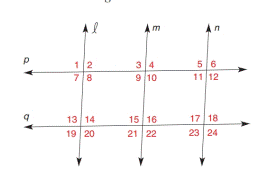Chapter 2.3, Problem 10EElementary Geometry For College St...

7th Edition
Alexander + 2 others
ISBN: 9781337614085

Solutions

Chapter
SectionElementary Geometry For College St...

7th Edition
Alexander + 2 others
ISBN: 9781337614085
Textbook Problem

In Exercise 7 to 16, name the lines (if any) that must be parallel under the given conditions.∠ 7 ≅ ∠ 11

To determine

To find:

The parallel lines.

Explanation

Given:

and n are cut by transversal p.

711

Approach:

If two lines are cut by a transversal so that two corresponding angles are congruent, then these lines are parallel.

Calculation:

The given statement is,

and n are cut by transversal p

Still sussing out bartleby?

Check out a sample textbook solution.

See a sample solution

The Solution to Your Study Problems

Bartleby provides explanations to thousands of textbook problems written by our experts, many with advanced degrees!

Get Started

Simplify: 1236

Elementary Technical Mathematics

Find the limit. limu1u41u3+5u26u

Single Variable Calculus: Early Transcendentals

True or False: A straight (simple) substitution may be used to evaluate 2+lnxxdx.

Study Guide for Stewart's Single Variable Calculus: Early Transcendentals, 8th